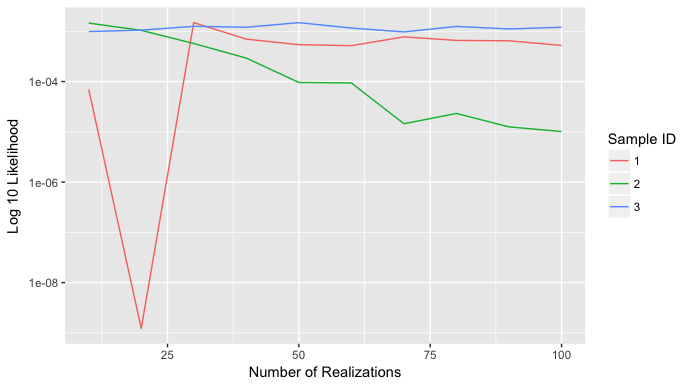# anchoredDistr

#### Mon Jun 19 2017

The anchoredDistr package is intended to handle the post-processing of projects created by MAD#, the software implmentation of the Method of Anchored distributions (see our Codeplex site). Similarly structured data not extracted from the MAD# software can also be used with some formatting.

However, the package is in early development and as such only has the following features:

• Reading the MAD# databases into a MADproject S4 class object
• Calculating non-parametric likelihood values using the np package
• Testing convergence of likelihood values as a function of the number of realizations
• Calculating posterior distributions
• Generating plots for the observations, realizations, and posteriors

while making the following assumptions:

• There is either multiple measurement locations with no time dependence or only one measurement location with a time series of data.
• The inversion data supported consist of the multi-well measurements, a subset of timesteps from the single measurement location’s time series, a parameter-less function (e.g. min) of that time series, or a function to be fitted to the time series (e.g. matern).

## Vignette Info

This vignette will step through an example of applying anchoredDistr using the dataset pumping, which contains results from a MAD# project pertaining to characterizing an aquifer’s mean natural-log hydraulic conductivity by using a time series of drawdown (change in hydraulic head) at a monitoring well in the field as inversion data. The MADproject object has slots numSamples, numAnchors, numTheta, observations, priors, true values, numLocations and realizations filled. Normally, the function readMAD would be used to fill in the object from databases produced by MAD#, but this has done already for pumping for a more portable example. However, MAD# is not entirely necessary: you can fill in a MADproject object with data from another application and still apply the MAD analysis.

For example, we can install and load the package:

install.packages(anchoredDistr)
library(anchoredDistr)

and then create a MADproject object given the following minimum information:

• Observations of inversion data: For the pumping example, there is one measurement location with 100 time steps. The format required is a vector of length (number of time steps).

• Prior distribution samples: The samples of the prior distributions for each strucutural parameter and anchor used. For the pumping example, there are 50 samples of one parameter and no anchors. The required format is a data.frame with columns sid (the sample ID), priordens (the marginal density associated with the sample), tid (the parameter ID), name (the parameter name), and priorvalue (the sampled value of the parameter).

• Realizations: Simulated values of the inversion data based on each of the prior samples. For the pumping example. The required format is a data.frame with columns sid (the sample ID), rid (the realization ID), zid (the inversion data ID), and value (the simulated value). Below shows the configuration of raw data in the pumpingInput data set and how it can be used to create a MADproject object without a MAD# database.

load(system.file("extdata", "pumpingInput.RData", package = "anchoredDistr"))
head(obs)
##    0.000000  -6.771288 -16.222989 -25.283413 -32.980097 -39.244209
head(realizations)
##        sid rid zid      value
## 200001   1   1   1  -8.378644
## 200002   1   2   1  -8.569131
## 200003   1   3   1  -8.720720
## 200004   1   4   1 -11.672956
## 200005   1   5   1  -9.151426
## 200006   1   6   1  -8.188874
head(priors)
##   sid priordens tid name priorvalue
## 2   1    0.3333   1 Mean       -9.0
## 3   2    0.3333   1 Mean       -9.5
## 4   3    0.3333   1 Mean      -10.0
proj <- new("MADproject",
numLocations = 1,
numTimesteps = 100,
numSamples   = 50,
numAnchors = 0,
numTheta = 1,
observations = obs,
realizations = realizations,
priors = priors)

However, the same data, plus more information, is available in the pumping dataset so it will be used for the remainder of the vignette.

data(pumping)

## Printing MADproject information

You can use the print function to preview what the MADproject object contains:

print(pumping)
## NAMES:
## The MAD project name: pumping
## The MAD result name: example2
## The path for the MAD project:
##
## CONFIGURATION VALUES:
## The number of measurement locations: 1
## The number of time steps: 100
## The number of samples: 3
## The number of anchors: 0
## The number of structural parameters: 1
##
## DATA DIMENSIONS:
## Size of true values: 1 2
## Size of observations: 100
## Size of realizations: 30000 4
## Size of priors: 3 5
## Size of likelihoods: 0 0
## Size of posteriors: 0 0

## Viewing Data

The plotMAD function can be used to view different data from the MADproject object. Pass the object as the first argument followed by

• nothing: yields all available plots given data
• "observations": yields a plot of the observation as a function of time step.
• "realizations": yields a plot of the samples’ realizations as a polygon representing the interquartile ranges of the realization values as a function of the time steps. Only works if @numSamples is less than six. The observations is also plotted for comparison.
• "posterior": yields the marginal posterior distributions for the samples.
• "prior": yields the marginal prior distributions for the samples.

Below is an example of requesting to plot the realizations for the pumping dataset.

plotMAD(pumping, "realizations")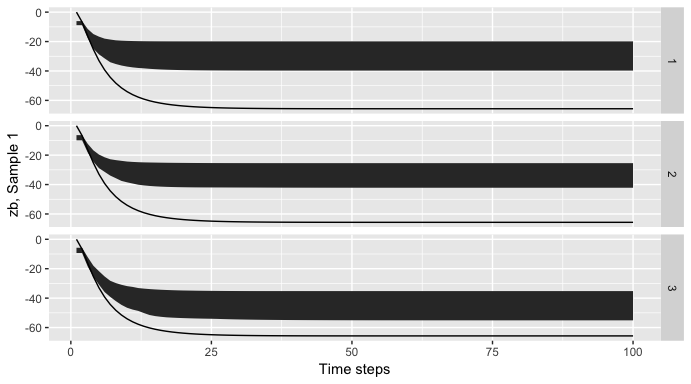## Applying MAD

The anchoredDistr package can take this information and calculate the posterior of the random parameter in question based on requested inversion data. Below, the 100th time step is used as the inversion data, then the posterior is calculated and plotted (again, using the plotMAD function).

pumping <- calcLikelihood(pumping, 100)
pumping <- calcPosterior(pumping)
plotMAD(pumping, "posteriors")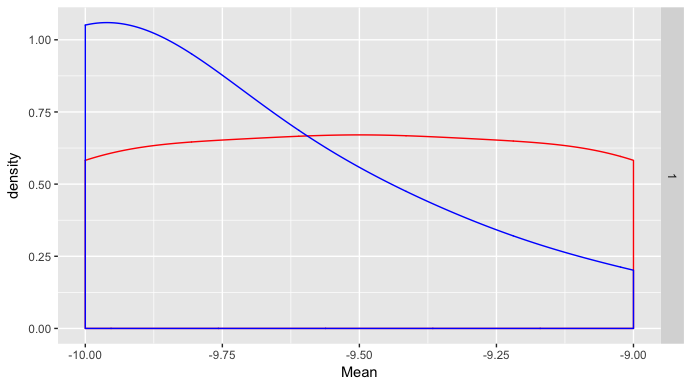## Applying MAD with Dimension Reduction

The anchoredDistr package can take this information and calculate the posterior of the random parameter in question based on requested inversion data. Below, the minimum value in the time series is used as the inversion data, then the posterior is calculated and plotted (again, using the plotMAD function). This is the same as using the 100th time step, but showcasing the ability to provide a function instead of a subset for the reduction.

pumping.min <- reduceData(pumping, min)
pumping.min <- calcLikelihood(pumping.min)
pumping.min <- calcPosterior(pumping.min)
plotMAD(pumping.min, "posteriors")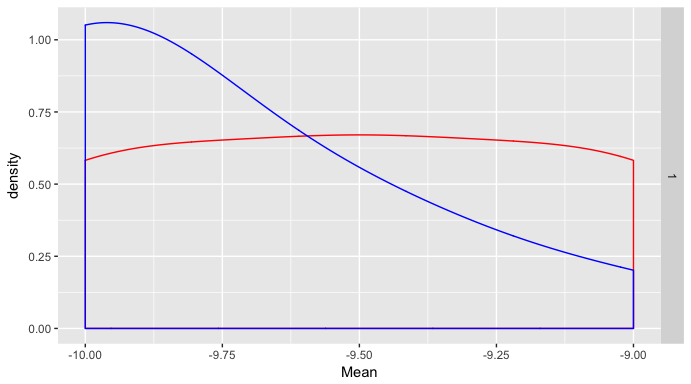Even more complicated functions can be passed. For example, this matern function:

matern <- function(x, params){
sigma <- params
lambda <- params
kappa <- params
t <- sqrt(2*kappa)*x/lambda
cov <-  ((sigma*(t^kappa)/gamma(kappa))*2^(1-kappa))*besselK(t,kappa)
return(sigma-cov)
}

If we want to fit this matern function to the time series, we need to provide nls with initial values for the three parameters. Here is a function to estimate these initial values:

init.matern <- function(x){
params<- c()
params <- min(x)
params <- min(10, tail(which(x > 0.3*min(x)),1))
params <- 0.5
return(params)
}

We can pass these two functions to reduceData for fitting a matern model to each time series and performing the inversion with the three parameters:

pumping.matern <- reduceData(pumping, matern, init.matern, lower=c(-Inf,1,0.1), upper=c(0,100,5), algorithm="port")
plotMAD(pumping.matern, "realizations")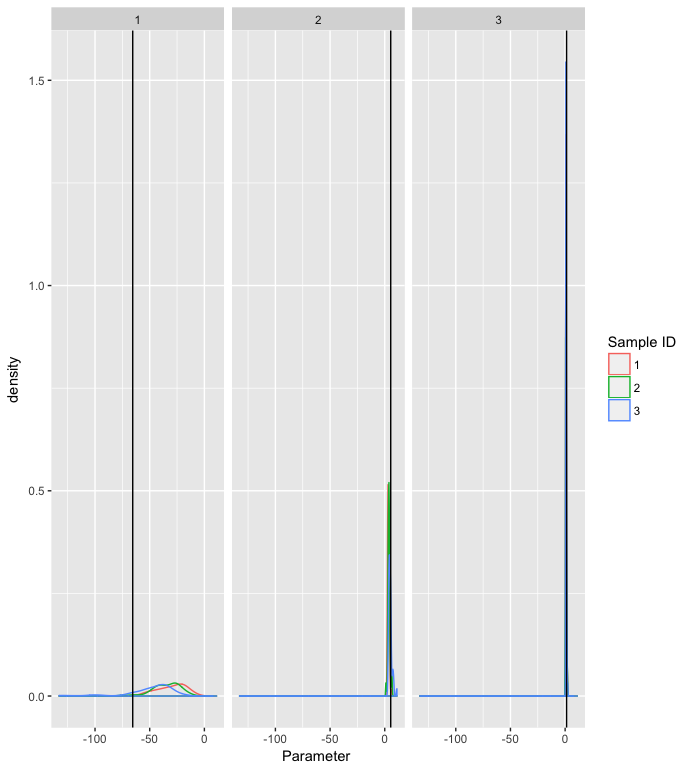pumping.matern <- calcLikelihood(pumping.matern)
pumping.matern <- calcPosterior(pumping.matern)
plotMAD(pumping.matern, "posteriors")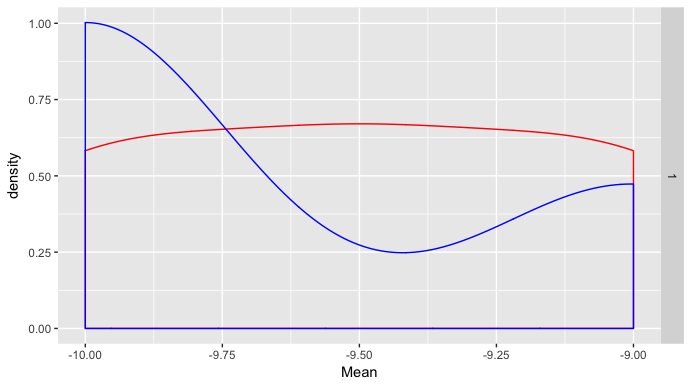## Convergence testing

In order to assess the convergence of the likelihood values, you can call the testConvergence function that will take a MADproject object and calculate likelihood values for a range of realization counts.

testConvergence(pumping.matern)